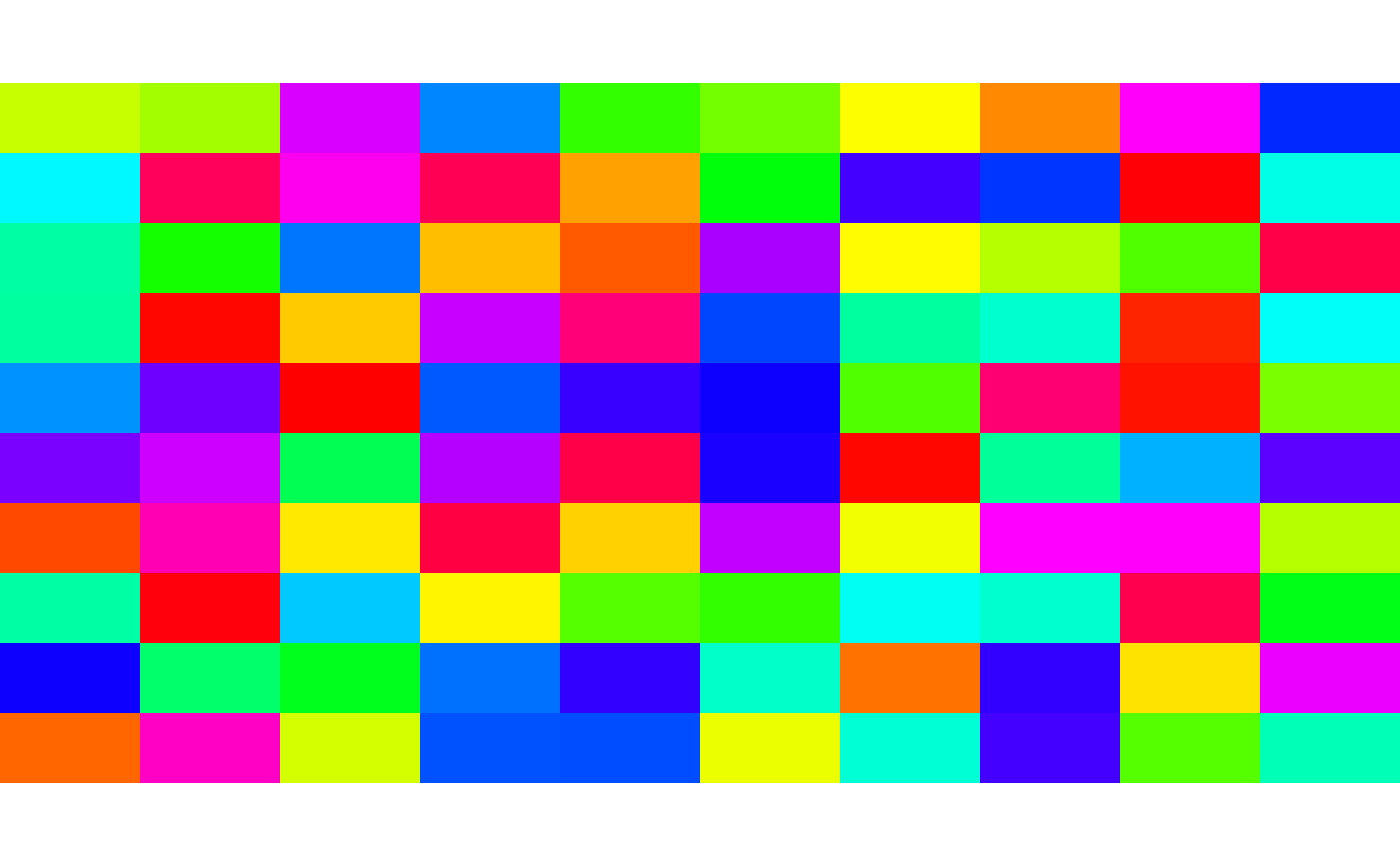Get or set the colortable of a RasterLayer. A colortable is a vector of 256 colors in the RGB triple format as returned by the rgb function (e.g. "#C4CDDA").

When setting the colortable, it is assumed that the values are integers in the range [0,255]

colortable(x)
colortable(x) <- value

## Arguments

x

RasterLayer object

value

vector of 256 character values

plotRGB

## Examples

r <- raster(ncol=10, nrow=10)
values(r) <- sample(0:255, ncell(r), replace=TRUE)
ctab <- sample(rainbow(256))
colortable(r) <- ctab
plot(r)